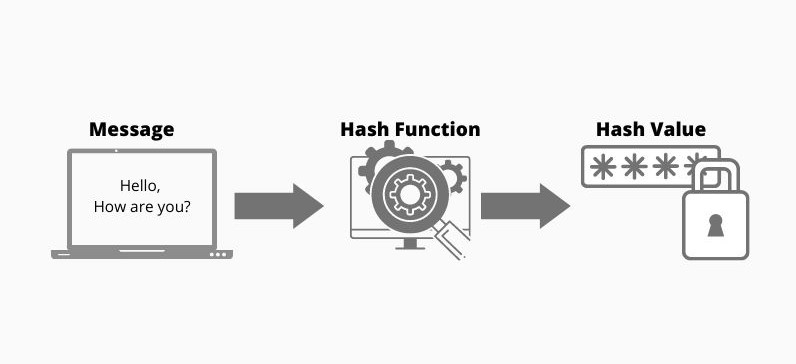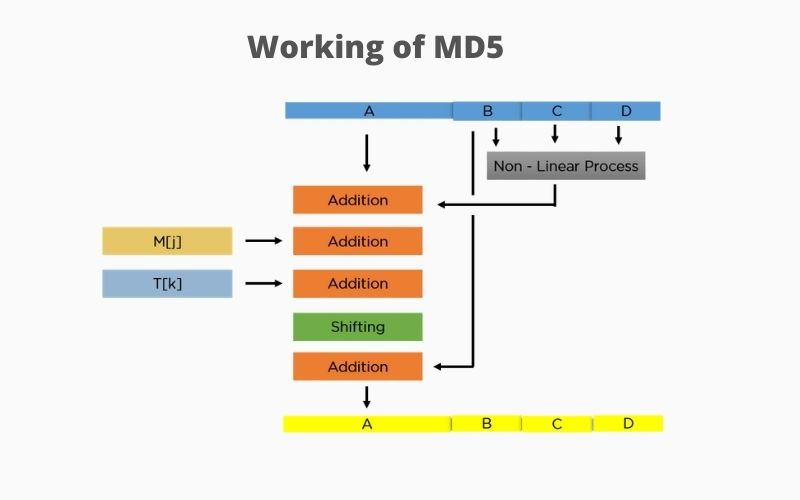# Get the MD5 Hash of a File in C++

MD5 is a cryptographic protocol that was previously used for encryption but now it is commonly used for authentication. It is based on the hashing process in the `hash` function that generates an encrypted hash value against some plain text.

## What Is the `hash` Function

Understanding the `hash` function is important before exploring the MD5 (Message-digest algorithm). Hashing is the `hash` function process used to convert plain text into some ciphertext with the hash value.

It is based on a mathematical function that helps convert plain text into ciphertext and generally a compressed hash value.This diagram demonstrates the working of the `hash` function; we provide some basic message that the message is an input to the `hash` function. It does some processes and gives us the output as an encrypted random value known as ciphertext.

### Features of `hash` Function

1. The output is always of the same length, 128 bits, without depending on the size of plain text.
2. It compresses the original message even if it is more than 128 bits.
3. It digests the data (Message), representing the data into a smaller hash value representation.
4. Hash values should be unique for every different message.
5. The hash value should always be the same for the same message.

## What Is MD5

The MD5 (Message-digest algorithm) is a cryptographic protocol used for authenticating a message, content verification and digital signatures; Ronald Rivest designed it in 1991 as an advanced version of MD4. It is based on the `hash` function to verify the sent and received files.

MD5 is now used for data authentication, but initially, it was used for data encryption. Authentication is one of the core properties of encryption which helps us in authentication and achieving data integrity.

MD5 is an efficient algorithm for the authenticity of passwords or verifying the originality of a file because it checks the hash values of files or passwords bit by bit.

There are 4 prominent steps of the MD5 algorithm:

2. Append length
3. Initialize MB buffer
4. Process each block

Our initial message can be of any size, 4000 bits, 1231 bits or any other number of bits; then, we add some padding bits to it. Lastly, you need to make sure the size of 64 bits is multiple of 512.

We add 1 at the beginning and the rest 0’s in padding.

### Append Length

In this step, to make your final message a multiple of 512, you need to add a few more characters to it. To do so, take the length of the original message and express it in the form of 64 bits.

And this combination gives us the final message which reading to be hashed.

### Initialize MB Buffer

It’s time to initialize the buffers `A`, `B`, `C`, and `D`; each buffer is used to compute the values for the message digest. Each buffer is 32 bits and initialized as follows:

``````A = 01 23 45 67
B = 89 ab cd ef
C = fe dc ba 98
D = 76 54 32 10
``````

### Process Each Block

Each 512 bits block is broken into further small chunks of 16 blocks; the size of each sub-block is 32 bits. Overall, four rounds process each block and perform some specific operations.

Each round utilizes all 16 blocks, and the buffers are constant array values.

Constant array is denoted as `T -> T`, and all sub-blocks are denoted as `M -> m`.According to this diagram, you can see that the values are being run for every single buffer.

``````void print_MD5(unsigned char* md, long size = MD5_DIGEST_LENGTH) {
for (int i=0; i<size; i++) {
cout<< hex << setw(2) << setfill('0') << (int) md[i];
}
}
``````

This function is used to print `MD` correctly.

``````#include <iostream>
#include "md5.h" // This is an external library that you need to import for MD5 algorithm

using namespace std; // for cout
int main(){
cout << "md5 of 'grape' : " << md5("grape") << endl;
return 0;
}
``````

Output:

``````md5 of 'grape' : b781cbb29054db12f88f08c6e161c199
``````

This code includes an external library, `md5.h`, allowing you to use the MD5 algorithm and generate hash values of plain text. We pass a string `grape` as an argument to the `md5` function that returns the file’s hash value.

``````fileSize = file.tellg();
cout << "File size \t"<< fileSize << endl;
memBlock = new char[fileSize];
file.seekg(0,ios::beg);
And at the end, we get compressing 128-bit value against the text. The MD5 algorithm hash value for the alphabet `a` looks like `0cc175b9c0f1b6a831c399e269772661`.